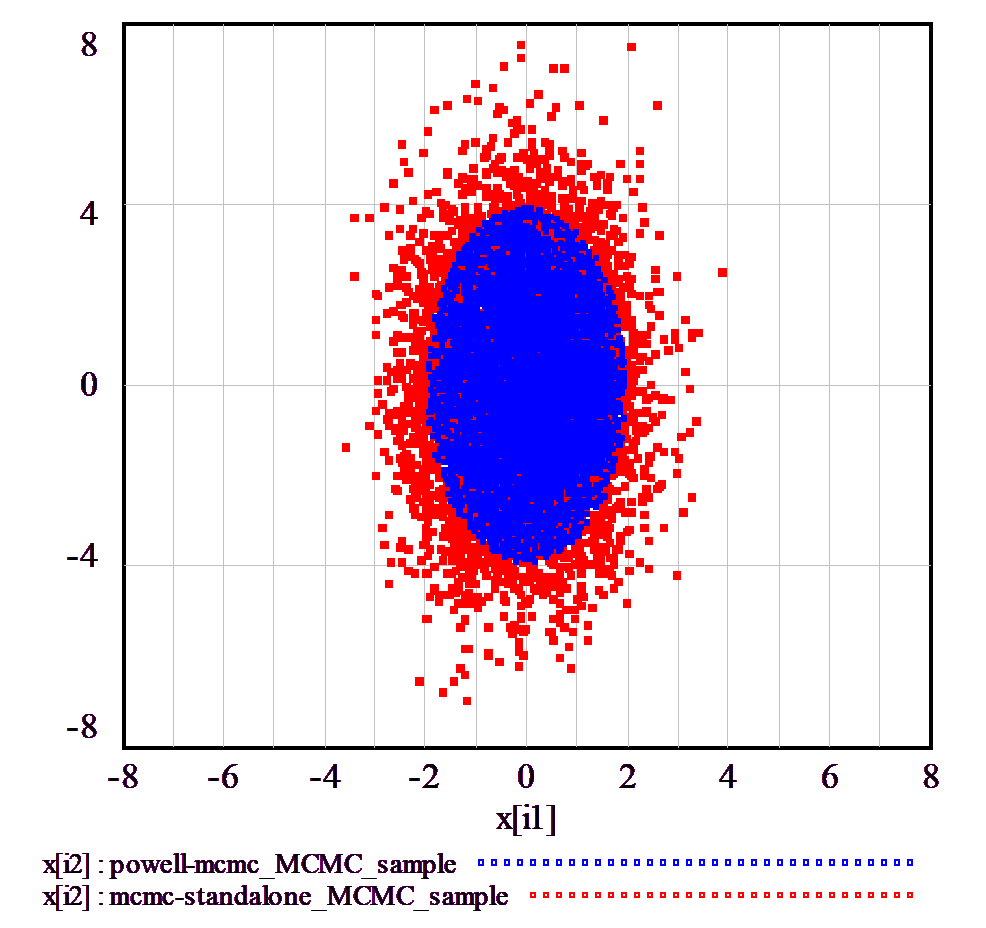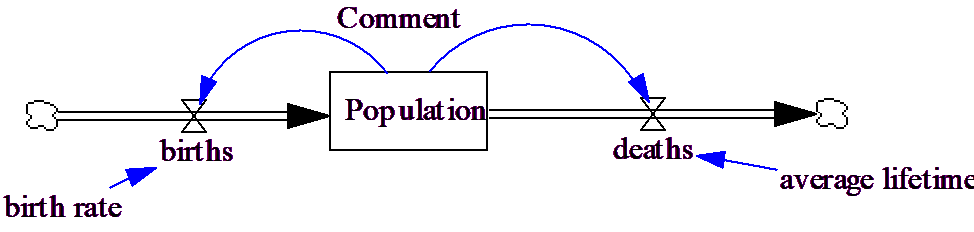# Vensim Help

 MCMC Output

The MCMC/SA algorithm can produce four kinds of output:

a sample of accepted points,

a diagnostic table of all points (except those that are rejected),

statistics of chain values and convergence, and

(when used as a payoff sensitivity method), confidence bounds on parameters.

All of these files can be imported to .vdf format for viewing in the model. The .tab files are convenient for reviewing in a spreadsheet. The points and statistics files contain additional variables, which can be seen by opening the .vdf as a model.

If you run very long simulations with many parameters, these files may grow very large.

Sample

A tab-delimited file, named myrun_MCMC_sample.tab, listing all accepted points and payoffs (after the optional burnin period). You may use it as a File method input to sensitivity runs, so that you can explore the response of variables in your model subject to the posterior probability from the calibration.

Note that MCMC does not accept a new point every iteration, so repeated points are common. These are important to the  statistical properties of the sample, but if you are using MCMC heuristically, you can suppress repeats with the MCRECORD option.

If you don't want to waste subsequent simulations on identical samples, you can generate a large sample with MCMC, then downsample it to a smaller set, which will have a low probability of repeats.

 varname 1 varname 2 varname 3 varname 4 # # # # # # # ...

The following example shows the sample resulting from standalone MCMC (red) and payoff sensitivity MCMC (blue). In the case of payoff sensitivity, the sample is truncated at the 95% confidence level, showing the characteristic elliptical shape of this bivariate normal distribution.The standalone MCMC sample has the mean and variance of the target distribution, including some points (not coincidentally, about 5%) that lie outside the 2-sigma 95% confidence bounds. The payoff sensitivity MCMC sample is the same inside the 95% bounds, but is truncated, so its total variance is less.

Points

A tab-delimited file, named myrun_MCMC_points.tab, listing all accepted points and payoffs.

 Iteration Payoff Chain Status varname 1 varname 2 varname 3 varname 4 1 # 1 0 # # # # 2 # 2 0 # # # ...

Status codes are as follows:

 -2 Payoff error (e.g., an FP error or negative payoff that should represent a likelihood)
 -1 Rejected
 0 Initial
 1 Accepted
 2 Repeated
 3 Accepted during burnin
 4 Repeated during burnin
 5 Accepted, but above payoff sensitivity threshold
 6 Repeated, but above payoff sensitivity threshold
 7 Improvement on best payoff (this will duplicate a point reported as 0-6)

Statistics

A .dat file, named myrun_MCMC_stats.dat, containing additional diagnostics. It includes:

Chain acceptance rates.

Flags for outlier chains.

Global mean and variance across all chains.

Global acceptance rate. The optimal acceptance rate for Normal distributions is about .24 under some conditions, though this is unlikely to hold in most models. An acceptance rate near 0 or 1 likely means that the proposal distribution is not generating viable points, or is too conservative, respectively. Either way, progress will be slow.

Best payoffs and improvements.

The grand mean and variance between chains, and the mean of chain variances over iterations.

The univariate Rubin/Brooks-Gelman PSRF convergence statistic. This compares variance across chains and within chains over iterations to indicate the extent to which additional iterations might improve the ratio of variances. PSRF should approach 1; values in excess of 1.2 are typically regarded as unconverged. Typically, the number of parallel chains in a simulation is large (at least 10, possibly 100s), so these are aggregated into a smaller set of metachains before the PSRF is computed.

A CMCP convergence statistic. This is a modification of the Rosenbaum cross-match permutation test. The value reported is a P-value of a Χ2 test for the uniformity of minimum distances for members within and between a current sample and a stored sample of points. At convergence, this should be uniformly distributed, and pass (exceed 5%) 95% of the time. The CMCP sample includes only accepted points, not repeats, so it may be misleading if the acceptance rate is varying substantially.

The number of parameter dimensions that may have collapsed variance (indicated by 0 interquartile range)

Statistical counters are reset at the end of burnin. Chain statistics are reset whenever an outlier chain is restarted. Means and variances are calculated via an online update that favors the second half of the available output (i.e., it is an exponentially weighted moving average).

The following shows healthy convergence, with PSRF close to 1, a reasonable acceptance rate (about .3), and CMCP seldom < .05.Stephen P. Brooks; Andrew Gelman, Journal of Computational and Graphical Statistics, Vol. 7, No. 4. (Dec., 1998), pp. 434-455.

Rosenbaum, P.R. (2005). An exact distribution-free test comparing two multivariate distributions based on adjacency. Journal of the Royal Statistical Society: Series B, 67, 515-530.

Payoff Sensitivity (Confidence) Bounds

(Payoff sensitivity only) a tab-delimited file, named myrun_sensitive.tab, listing parameter confidence bounds.